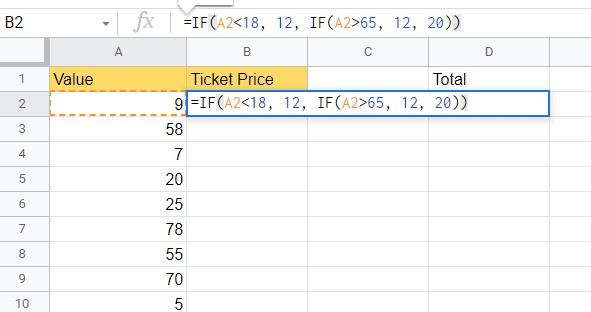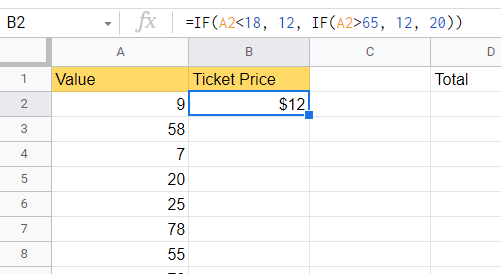# How to Use If and Nested If in Google Sheets

Learning how to use nested IF functions in Google Sheets is useful when you need to add more complex logic to your spreadsheet.

Nested IFs refer to formulas that include IF statements inside other IF statements. This structure allows us to come up with more complex checks.

The IF function is useful when you want data in a certain cell to depend on whether a certain conditional statement is True or False. Nesting multiple `IF` functions together allows us to make multiple conditional checks in a single formula.

Let’s take a look at a scenario where we can use nested `IF` functions.

We have a list of museum attendants, and we’d like to figure out how much to charge them per ticket. We want to charge people below 18 and senior citizens with a \$12 ticket. Regular admission will be priced at \$20. How can we do this?

It becomes quite easy to recreate this logic on a spreadsheet with nested `IF` functions. We can have an `IF` statement that checks if the attendant’s age is below 18 and another that checks if they are above 65.

This use case is just one way to use nested `IF` functions in Google Sheets. Now that we know when to use them, let’s learn how to write nested `IF` functions on an actual sample spreadsheet.

## A Real Example of Nested IF Functions in Google Sheets

Let’s look at a real example of nested `IF` functions used in Google Sheets spreadsheet.

Below is a list of 25 ticket holders and their corresponding ages. We used the `IF` statement to compute the ticket price in column B. If the ticket holder is less than 18 years of age, they’ll be charged \$12, and if they’re 18 and above, they’ll be charged \$20.To get the values in Column B, we just need to use the following formula:

`=IF(A2<18,12,20)`

What if we want to also give a discount to senior citizens? We can give the formula two conditional checks using a nested `IF` statement. In the example below, we’ve added the rule that any ticket holder older than 65 will be charged \$12 per ticket.To get these values in Column B, we just need to use the following formula:

`=IF(A2<18,12, IF(A2<65,12,20))`

If you’re ready to try using nested IF functions in Google Sheets, let’s start writing it step-by-step!

## How to Use Nested IF Functions in Google Sheets

This section will go through each step needed to start using nested IFs in Google Sheets. This guide will show you how to use multiple conditionals to apply complex logic to your worksheet.

Follow these steps to start using nested `IF` functions:

1. First, select the cell that will hold the result of our `IF` statement. In this example, we’ll start with cell B2.2. Next, we just simply type the equal sign ‘=‘ to begin the function, followed by ‘IF(‘.
3. You may find a tooltip box with hints on using the `IF` function. Click on the arrow found on the top-right-hand corner to minimize it if needed.4. Next, we need to write the first conditional to check. In our example, we’ll first do a check if our value in column A is less than 18.5. The second argument will be the value of the ticket if the first argument is True. If the value of A2 is less than 18, then the formula will evaluate to \$12.6. Next, we’ll write the nested IF in our third argument. Similarly, this nested `IF` checks the value in column A for a specific condition. In this example, we want to check if their age is over 65.7. For the last argument in the nested `IF` function, we add the price if we get False in the previous two checks. If our attendants are neither below 18 or above 65, they’ll pay for a \$20 ticket.8. Lastly, we drag down the formula to fill out the rest of the column.1. Is there an easier way to write out nested IF functions?
Writing long nested IFs might become difficult to maintain or understand. You can look into trying the `IFS` function to evaluate a set of expressions without using any nested IFs.

With the IFS function we convert something like `=IF(A2<18, 12, IF(A2>65, 12, 20))` into `=IFS(A2<18,12,A2>65,12,A2<=65,20)`

That’s all you need to remember to start using nested IFs in Google Sheets. This step-by-step guide shows how easy it is to use multiple conditionals to create complex logical rules for your spreadsheet.

You can use Nested IF functions with other Google Sheets functions to create powerful spreadsheets.

Are you interested in learning more about what Google Sheets can do? Subscribe to our newsletter to be the first to know about the latest Google Sheets guides and tutorials from us.Our goal this year is to create lots of rich, bite-sized tutorials for Google Sheets users like you. If you liked this one, you'd love what we are working on! Readers receive ✨ early access ✨ to new content.

##### You May Also Like## How to Use the COUPNUM Function in Google Sheets

The COUPNUM function in Google Sheets is used to calculate the number of coupons (interest payments) that will…## How to Convert Month Name to Number in Google Sheets

Knowing how to convert month name to number in Google Sheets is useful if you have your month…## How to Use DOLLARFR Function in Google Sheets

The DOLLARFR function in Google Sheets is useful if you want to convert a price given as a…## How to Use STDEVP Function in Google Sheets

The STDEVP function in Google Sheets is useful to calculate the standard deviation across an entire population. It…